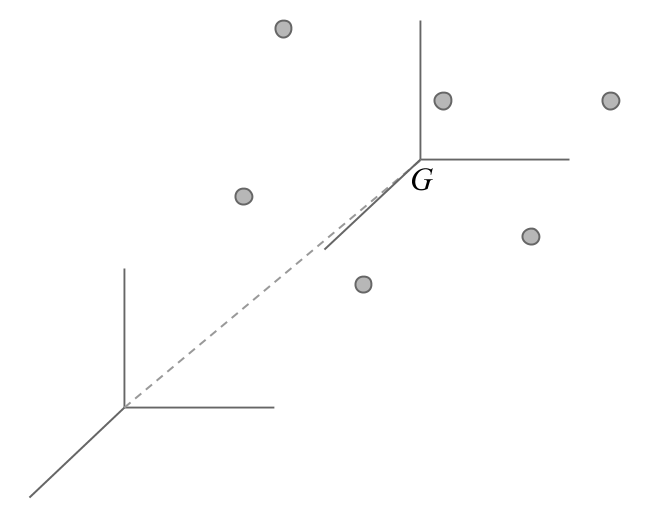5.6 Centered and Standardized Variable

We say that a variable is mean-centered when its mean is zero. When we centered all the variables, we shift the origin of a cloud of points to the center of gravity.In addition, if we divide each value by the standard deviation of the variable, this becomes mean-centered and standardized. The variance of a centered and standardized variable is equal to one.

\begin{align*} z_{ij} &= \frac{x_{ij} - \bar{x}_j}{s_j} \\ & \\ var(\mathbf{z_j}) &= \sum_{i=1}^{n} p_i (z_{ij} - \bar{z}_j)^2 = \frac{\sum_{i=1}^{n} p_i (x_{ij} - \bar{x}_j)^2}{s_{j}^{2}} = 1 \end{align*}

The variance of a variable is the squared of the distance from the variable-point to the origin. The centered and standardized variables have a distance of one unit from the origin (i.e. they are on a sphere of radius 1).